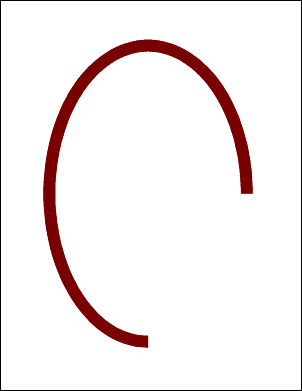AddArc FunctionAdds an arc to the current page.Syntax

 [C#] ```int AddArc(double as, double ae, double cx, double cy, double rx, double ry) int AddArc(double as, double ae, double cx, double cy, double rx, double ry, bool filled)``` [Visual Basic] ```Function AddArc(as As Double, ae As Double, cx As Double, cy As Double, rx As Double, ry As Double) As Integer Function AddArc(as As Double, ae As Double, cx As Double, cy As Double, rx As Double, ry As Double, filled As Boolean) As Integer```Params

 Name Description as The start angle of the arc in degrees. ae The end angle of the arc in degrees. cx The horizontal center of the arc. cy The vertical center of the arc. rx The horizontal radius of the arc. ry The vertical radius of the arc. filled Whether to fill the arc rather than simply drawing it. return The Object ID of the newly added Graphic Object.Notes

 Adds an arc to the current page. The arc is drawn in the current color at the current width and with the current options. The arc is fixed at the center coordinate and can have different horizontal and vertical radii. Drawing starts at the start angle and the arc is swept out until the end angle is reached. Angles are measured anti-clockwise with zero at three o'clock. The AddArc function returns the Object ID of the newly added Graphic Object.Example

 The following code adds an arc to a document.   ```using (Doc doc = new Doc()) {   doc.Width = 24;   doc.Color.String = "120 0 0";   doc.AddArc(0, 270, 300, 400, 200, 300);   doc.Save(Server.MapPath("docaddarc.pdf")); } ``` ```Using doc As New Doc()   doc.Width = 24   doc.Color.String = "120 0 0"   doc.AddArc(0, 270, 300, 400, 200, 300)   doc.Save(Server.MapPath("docaddarc.pdf")) End Using ```docaddarc.pdf